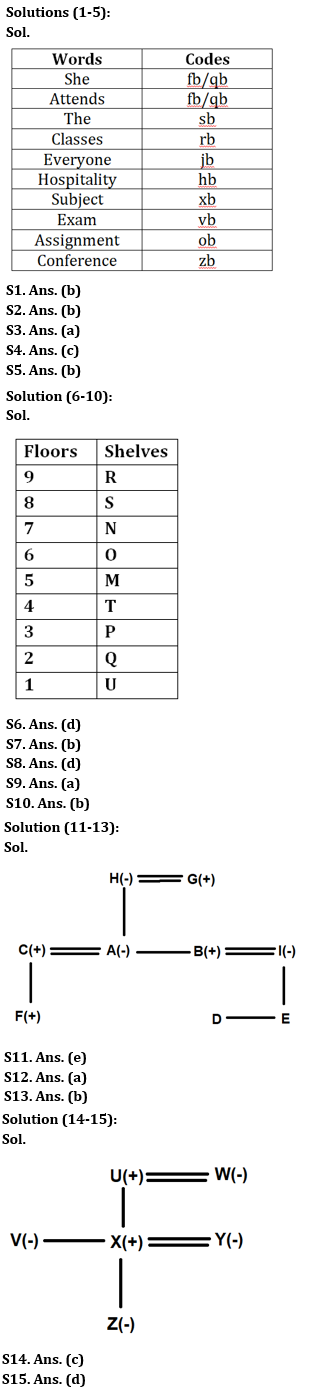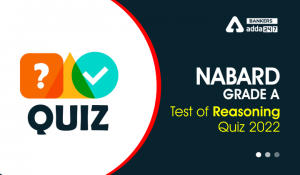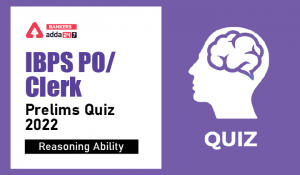Latest Banking jobs   »

# Reasoning Ability Quiz For IBPS RRB PO Clerk Prelims 2022- 7th July

Directions (1-5): Answer the questions based on the information given below.
In a certain language,
“She attends the classes everyone” is coded as “sb rb fb jb qb”
“The hospitality subject exam” is coded as “hb vb sb xb”
“Assignment exam everyone” is coded as “jb ob vb”
“She attends hospitality conference” is coded as “qb fb zb hb”

Q1. What is the code for ‘subject’?
(a) hb
(b) xb
(c) vb
(d) qb
(e) None of these

Q2. Which of the following word is coded as ‘zb’?
(a) Everyone
(b) Conference
(c) She
(d) The
(e) None of these

Q3. What is the code for ‘assignment’?
(a) ob
(b) qb
(c) vb
(d) sb
(e) Can’t be determined

Q4. What is the code for ‘attends’?
(a) fb
(b) qb
(c) Either ‘fb’ or ‘qb’
(d) zb
(e) Either ‘qb’ or ‘zb’

Q5. What is the code for ‘classes’?
(a) sb
(b) rb
(c) fb
(d) qb
(e) Can’t be determined

Directions (6-10): Answer the questions based on the information given below.
There are 9 shelves M, N, O, P, Q, R, S, T and U in a cupboard. The bottommost shelf is numbered as 1, just above it is numbered as 2 and so on till the topmost shelf is numbered as 9. There are two shelves between shelf N and shelf T. Shelf N is five shelves above shelf Q which is a prime numbered shelf. There is one shelf between shelf T and shelf O. Shelf R is just above shelf S. Shelf M is above shelf P which is above shelf U. M is not the topmost shelf.

Q6. How many shelves are above shelf M?
(a) Three
(b) Five
(c) Two
(d) Four
(e) Six

Q7. Which of the following is topmost self?
(a) T
(b) R
(c) O
(d) N
(e) U

Q8. Shelf___ is just above shelf P.
(a) S
(b) N
(c) O
(d) T
(e) M

Q9. How many shelves are between shelf O and shelf Q?
(a) Three
(b) Five
(c) One
(d) Four
(e) Six

Q10. Four of the five are related to each other in some way and thus form a group. Choose the one which does not belong to that group.
(a) S
(b) P
(c) O
(d) T
(e) Q

Directions (11-13): Answer the questions based on the information given below.
Nine persons A, B, C, D, E, F, G, H & I are members of a family consisting of three generations and three married couples. I is the only sister- in- law of A who is only daughter of H. Only A and B are children of G. F is son of C. D and E are children of I. G is paternal grandfather of E.

Q11. How many Female members are there in the family?
(a) Three
(b) Four
(c) Five
(d) Six
(e) Cannot be determined

Q12. Who is the paternal aunt of D?
(a) A
(b) B
(c) H
(d) G
(e) None of the above

Q13. How is C related to son of H?
(a) Sister- in- law
(b) Brother- in -law
(c) Brother
(d) Sister
(e) None of the above

Directions (14-15): Answer the questions based on the information given below.
There are six members U, V, W, X, Y & Z in the family. There is no single parent in the family. V is the sister of Z’s father. Z is the only granddaughter of U. Y is the only daughter-in-law of W who is not the father of V.

Q14. How is X related to U?
(a) Grandson
(b) Granddaughter
(c) Son
(d) Daughter
(e) None of the above

Q15. How many female members are there in the family?
(a) One
(b) Two
(c) Three
(d) Four
(e) None of the above

Solutions#### Congratulations!Incorrect details? Fill the form again here

•Reasoning Ability Quiz For IBPS RRB PO C...
•Reasoning Ability Quiz For NABARD Grade ...
•Reasoning Ability Quiz For IBPS Clerk/PO...
•Reasoning Ability Quiz For IBPS RRB PO C...
•Reasoning Ability Quiz For IBPS RRB PO C...
•Reasoning Ability Quiz For IBPS Clerk Pr...